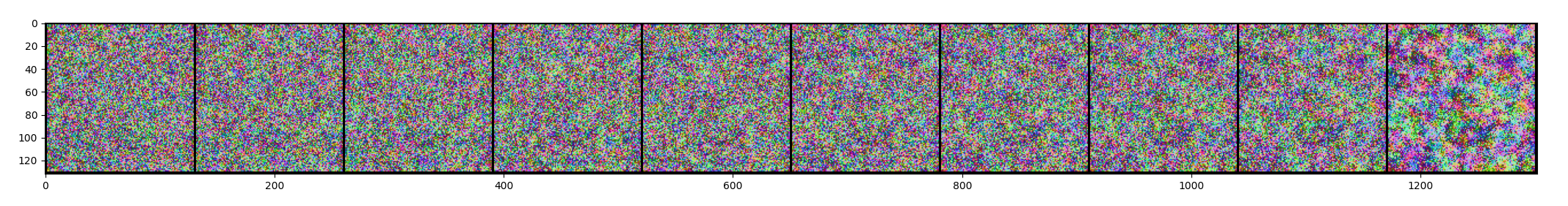## Motivation§

The current paradigm in diffusion models is to use a modified UNet, which are based on convolutional neural networks (CNNs). Unfortunately, convolutional layers are resolution-dependent, i.e. in image synthesis tasks, we cannot simply generate an arbitrarily sized image. There has been prior work conditional diffusion where we can extend images (à la OpenAI’s Dall-E 2 Outpainting).

## Idea§

Replace the UNet structure with an MLP that can predict individual pixel values of the reverse process. If successful, this makes the diffusion process resolution-independent.

Obviously, this task seems to require some sort of context or knowledge of surrounding pixels. To attempt to provide this, we try Gaussian blur to get context of surrounding pixels and pass that along with the pixel value and coordinate.

## Post mortem§

Unfortunately, this idea did not work – at least in the way that I tried.

The last MLP design I used was with 3 hidden layers that took in an input vector $$\mathbf{v} := \langle \vec{x}, t, \mathbf{I}_t(\vec{x}), \mathbf{N}_1(\vec{x}), \mathbf{N}_2(\vec{x}), \dots, \mathbf{N}_k(\vec{x})\rangle$$ where

• $\vec{x}$ are the $x$ and $y$ image coordinates,
• $t$ is the time step,
• $\mathbf{I}_t(\vec{x})$ are the RGB values of the image (after $t$ forward steps) at $\vec{x}$,
• $\mathbf{N}_1(\vec{x}), \mathbf{N}_2(\vec{x}), \dots, \mathbf{N}_k(\vec{x})$ are the original image $\mathbf{I}$ blurred using Gaussian blur with their RGB values sampled at $\vec{x}$,

(all components normalized to $[-1, 1]$).

In any case, I tried various activation functions, numbers of hidden layers, number of units in trying to get this coordinate diffusion MLP to generate something. In the end, all attempts ended up looking like noise – not like Gaussian noise (see below).The output of the network was simply $\mathbf{I}_{t-1}(\vec{x}),$ or the RGB values at pixel $\vec{x}$ at the previous time step.

It might be worth looking at other coordinate based MLPs that generate pixel values like:

It is worth noting that these architectures are trained on individual scenes, so maybe they won’t generalize to image synthesis in diffusion? Maybe an MLP with skip-connections might yield some result?